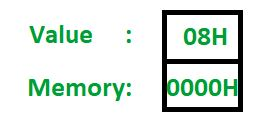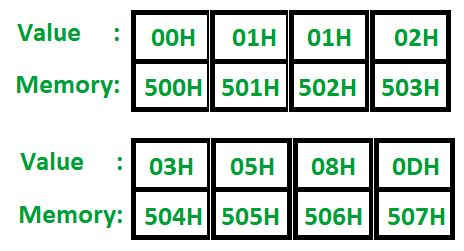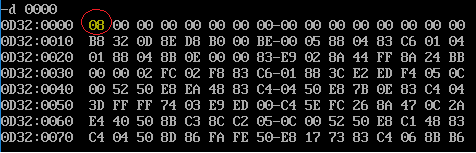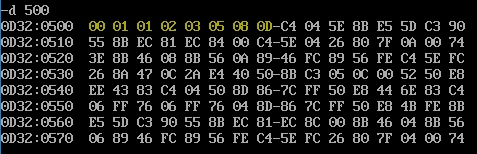# 8086 program to generate Fibonacci Sequence

• Last Updated : 22 Feb, 2019

Problem – Write a 8086 assembly level program to generate the Fibonacci Sequence. The length of the sequence is stored in the data segment with the offset value of 0. We will store the generated sequence in the data segment, from the offset value of 500.
Note:The generated numbers and memory locations are represented in their Hexa-Decimal format.

Example:
Input:

Attention reader! Don’t stop learning now. Get hold of all the important CS Theory concepts for SDE interviews with the CS Theory Course at a student-friendly price and become industry ready.Output:Algorithm:
The Fibonacci sequence is generated by adding the (i)th element and the (i-1)th element, and storing it into the (i+1)th position. This holds good given that the 1st and 2nd positions are initialized with 0 and 1 respectively. The following steps need to be followed to execute the process using the Assembly Level instructions.

1. Move the value stored at offset 00H into CX(this will act as the counter), and decrement it by 2 (because we need to explicitly load the first 2 elements of the sequence)
2. Move 00H into AL
3. Move 500 into SI
4. Move AL into [SI]
5. Increment both AL and SI by 1, and store AL’s value in [SI] (with this, we have loaded the first 2 elements of the sequence into the memory)
6. Move [SI-1]th value into AL
7. Move [SI]th value into AH
8. Move 00H into BH
9. Add BH and AH (result stored in BH)
10. Add BH again with AL
11. Increment SI by 1
12. Store BH into [SI]
13. Loop back to Step 6 till counter becomes 0
14. Stop

Program:

Instruction/MnemonicExplanation/Comment
2000MOV AL, 00H
AL <- 00
2002MOV SI, 500HSI <- 500
2005MOV [SI], AL[SI] <- 00
2007ADD SI, 01HSI <- SI + 01
200AADD AL, 01HAL <- AL + 01
200CMOV [SI], ALSI <- AL
200EMOV CX, [0000H]CX <- 0000
2012SUB CX, 0002HCX <- CX – 02
~2015L1:LABEL
2015MOV AL, [SI-1]AL <- [SI – 01]
2018ADD AL, [SI]AL <- AL + [SI]
201AADD SI, 01HSI <- SI + 01
201DMOV [SI], AL[SI] <- AL
201FLOOP L1LOOP L1 CX TIMES
2021HLTEND

Explanation:

1. MOV AL, 00H: AL now has the 1st number from the sequence
2. MOV SI, 500H: Making the SI point to the output location
3. MOV [SI], AL: Moving 0 into the first position
4. ADD SI, 1: Increment SI to point to the next memory location
5. ADD AL, 1: Now, AL has the 2nd element of the sequence
6. MOV [SI], AL: Moving 01H into the 2nd position
7. MOV CX, [0000H]: Moving the value stored at offset 0 into CX(counter)
8. SUB CX, 02H: Since we have initialised the first 2 elements of the sequence, we need to decrement the counter by 2
9. L1: This defines the start of the loop (A label is created)
10. MOV AL, [SI-1]: Moves the element in the (i-1)th position into AL
11. ADD AL, [SI]: Moves the (i)th element with the (i-1)th element already present in AL
12. ADD SI, 1: Increment SI to point to the next position
13. MOV [SI], AL: Store the sum in the new position
14. LOOP L1: The instructions between label L1 and this LOOP instruction are executed “CX” times
15. HLT: Ends the program

## Actual Output:

The circled memory location (0000) contains the length of the sequence. For this program, it is 8.The highlighted values are the elements of the Fibonacci Sequence (in their Hexa-Decimal representation. Hence, 13 is represented as 0D)Enjoy coding!

My Personal Notes arrow_drop_up